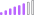cancel
Showing results for
Did you mean:Post Patron

## Extract numerous Key words from sentences in a cloumn

I have a description column that contains descriptions of items. These descriptions are in a sentence format and contain one of over 30  key words.  A few of the key words are below. I would like to extract the key words from the description. For Example in the table below the Description for row 1 would only show MATCode and exclude the rest of the sentence. How can I accomplish this? I am open to doing it as a measure or in the query editor. whichever is most effective because I have over 30 key words

Key Words

MATCode

LNCMCA

LMTDWN

DECWEEM@AEEG

DECEESM@HDDC

DEFFMM@LKKDDI

DSFDFMM@SSSS

NfdIS@CffffVID-19@AffS37F

NgfgDIS@gdgdVID-19@A01S37F

 PO Number Descripton 1 Truck Lift MATCode update 2 Data LNCMCA Review 3 Update LMTDWN Analysis Integrity 4 DECWEEM@AEEG Modification 5 Title Revised DECEESM@HDDC 6 System Processor Technology DEFFMM@LKKDDI 7 DSFDFMM@SSSS Update 8 NfdIS@CffffVID-19@AffS37F Technology 9 NgfgDIS@gdgdVID-19@A01S37F
1 ACCEPTED SOLUTIONSuper User

@dw700d This is a variation on Text to Table. PBIX is attached below sig.

``````Keyword =
VAR __Separator = " "
VAR __SearchText = MAX('Table'[Description])
VAR __Len = LEN(__SearchText)
VAR __Count = __Len - LEN(SUBSTITUTE(__SearchText,__Separator,"")) + 1
VAR __Table =
SELECTCOLUMNS(
GENERATESERIES(1,__Count,1),
"__Word",
VAR __Text = SUBSTITUTE(__SearchText,__Separator,"|",IF([Value]=1,1,[Value]-1))
VAR __Start =
SWITCH(TRUE(),
__Count = 1,1,
[Value] = 1,1,
FIND("|",__Text)+1
)
VAR __End =
SWITCH(TRUE(),
__Count = 1,__Len,
[Value] = 1,FIND("|",__Text) - 1,
[Value] = __Count,__Len,
FIND(__Separator,__Text,__Start)-1
)
VAR __Word = MID(__Text,__Start,__End - __Start + 1)
RETURN __Word
),
"__Word", [__Word]
)
VAR __Keywords = INTERSECT(__Table,SELECTCOLUMNS('Keywords',"__Word",[Key Words]))
RETURN
CONCATENATEX(__Keywords,[__Word],",")``````

Become an expert!: Enterprise DNA
External Tools: MSHGQM
Latest book!:
Mastering Power BI 2nd EditionDAX is easy, CALCULATE makes DAX hard...
6 REPLIES 6Super User

@dw700d This is a variation on Text to Table. PBIX is attached below sig.

``````Keyword =
VAR __Separator = " "
VAR __SearchText = MAX('Table'[Description])
VAR __Len = LEN(__SearchText)
VAR __Count = __Len - LEN(SUBSTITUTE(__SearchText,__Separator,"")) + 1
VAR __Table =
SELECTCOLUMNS(
GENERATESERIES(1,__Count,1),
"__Word",
VAR __Text = SUBSTITUTE(__SearchText,__Separator,"|",IF([Value]=1,1,[Value]-1))
VAR __Start =
SWITCH(TRUE(),
__Count = 1,1,
[Value] = 1,1,
FIND("|",__Text)+1
)
VAR __End =
SWITCH(TRUE(),
__Count = 1,__Len,
[Value] = 1,FIND("|",__Text) - 1,
[Value] = __Count,__Len,
FIND(__Separator,__Text,__Start)-1
)
VAR __Word = MID(__Text,__Start,__End - __Start + 1)
RETURN __Word
),
"__Word", [__Word]
)
VAR __Keywords = INTERSECT(__Table,SELECTCOLUMNS('Keywords',"__Word",[Key Words]))
RETURN
CONCATENATEX(__Keywords,[__Word],",")``````

Become an expert!: Enterprise DNA
External Tools: MSHGQM
Latest book!:
Mastering Power BI 2nd EditionDAX is easy, CALCULATE makes DAX hard...New Member

@Greg_Deckler Thank you for this solution. I've managed to adjust it to my table but now I get an expression error on line 32 not a valid table expression.

I'm sure I forgot a step just no idea what.

I tried opening your keywords.pbix but unfortunately we using an older version and I don't have permission to upgrade.Super User

@LeVa Hard to say, can you post your code?

Become an expert!: Enterprise DNA
External Tools: MSHGQM
Latest book!:
Mastering Power BI 2nd EditionDAX is easy, CALCULATE makes DAX hard...New Member

@Greg_Deckler I'm sorry I added a screenshot with the code, must have done something wrong there.

This is what I have now.

Keyword =
VAR __Separator = " "
VAR __SearchText = MAX('Servicedesk'[JobDescr])
VAR __Len = LEN(__SearchText)
VAR __Count = __Len - LEN(SUBSTITUTE(__SearchText,__Separator,"")) + 1
VAR __Table =
SELECTCOLUMNS(
GENERATESERIES(1,__Count,1),
"__Word",
VAR __Text = SUBSTITUTE(__SearchText,__Separator,"|",IF([Value]=1,1,[Value]-1))
VAR __Start =
SWITCH(TRUE(),
__Count = 1,1,
[Value] = 1,1,
FIND("|",__Text)+1
)
VAR __End =
SWITCH(TRUE(),
__Count = 1,__Len,
[Value] = 1,FIND("|",__Text) - 1,
[Value] = __Count,__Len,
FIND(__Separator,__Text,__Start)-1
)
VAR __Word = MID(__Text,__Start,__End - __Start + 1)
RETURN __Word
),
"__Word", [__word]
)
VAR __Keywords = INTERSECT(__Table,SELECTCOLUMNS('Servicedesk',"__Words", Servicedesk[JobDescr]))
RETURN
CONCATENATEX(__Keywords,[__Word],",")Post Patron

Thanks @Greg_Deckler for the prompt response I am not familiar with the text to table concept. What does this measure allow me to do?Super User

@dw700d It extracts the keywords from the sentences and lists them. You can see it in action here:

Keywords - Microsoft Power BI Community

Pretty sure it does exactly what you want to be done. Basically the way it works, it converts the sentence into a table of words. It then INTERSECTS the table of words with the table of keywords. It then concatenates the results.

Become an expert!: Enterprise DNA
External Tools: MSHGQM
Latest book!:
Mastering Power BI 2nd EditionDAX is easy, CALCULATE makes DAX hard...